Next: MINIMUM K-CLUSTERING Up: Miscellaneous Previous: MINIMUM BROADCAST TIME   Index

### MINIMUM K-CENTER

• INSTANCE: Complete graph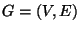and distancessatisfying the triangle inequality.

• SOLUTION: A k-center set, i.e., a subsetwith.

• MEASURE: The maximum distance from a vertex to its nearest center, i.e.,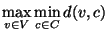.

• Good News: Approximable within 2  and .

• Bad News: Not approximable within 2for anyand .

• Comment: Not in APX if the distances do not satisfy the triangle inequality . MINIMUM CAPACITATED K-CENTER, the variation in which the number of vertices each center can serve is bounded by a constant L, is approximable within 5 . The converse problem, where the maximum distance from each vertex to its center is given and the number of centers is to be minimized, is approximable within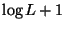. The geometric k-center problem, where the vertices lie in the plane and the geometric metric is used, is approximable within 2, but is not approximable within 1.822 . Variants of the geometric k-center problem are also studied in the paper. The rectilinear k-center problem, where the vertices lie in the plane and the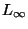metric is used, is approximable within 2, but is not approximable within 2for any. If the vertices lie in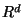and the Euclidean ormetric is used the problem admits a PTAS whose time is exponential in k . Approximable within 2 in any metric space .

The vertex weighted version, where the objective is to minimize the maximum weighted distance d(v,c)w(v), is approximable within 2 . It is not approximable within 2even if the distances are induced by a planar graph of maximum degree 3 with edge lengths 1 and vertex weights 1 . MINIMUM ABSOLUTE K-CENTER, the variation in which we allow the points in C to lie in edges (considered as curves) is also approximable within 2 and is not approximable within. MINIMUM -ALL-NEIGHBOR K-CENTER, the variation in which we want to minimize the distance from each vertex to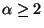of the k centers, is approximable within 2 whenand within 3 otherwise (even for the vertex weighted version) ; it is not approximable withinfor any. The asymmetric k-center problem, where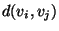might be different from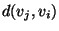, is approximable within.

• Garey and Johnson: Similar to ND50Next: MINIMUM K-CLUSTERING Up: Miscellaneous Previous: MINIMUM BROADCAST TIME   Index
Viggo Kann
2000-03-20